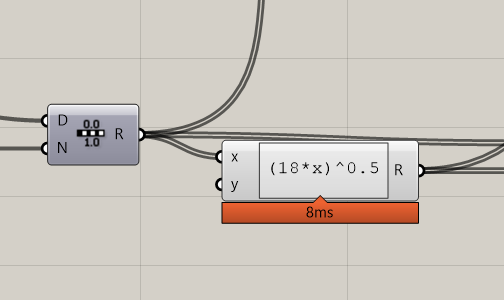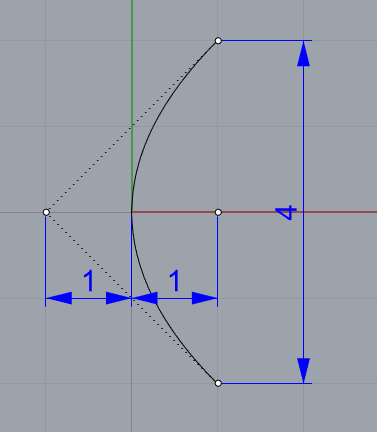# Equations for a Parabolic Curve

I am trying to model this shape that I have been given an equation for, but can not understand how to make the equations work in GH or if I am even doing this the best way.

My plan was to pass a range of X points into the equation to give a range of Y points, plot them, and join the dots.

Curve.gh (7.7 KB)its just the syntax

Yeah nice one, Ive also managed to do it using the multiply button within the equation editor thingy.

Curve3.gh (10.7 KB)

I have no idea if the sketch I was given is not accurate or if I have done something wrong… will have to find out, but at least i have a curve now!

the curve I got by using that equation is far off from the one presented in the initial image, and also far off in depth when width is 457.20 unitsis it supposed to be like that?

1 Like

Do the Math and always pays attention to units
18 inch = 457.2 mm

parabolic.gh (9.7 KB)

2 Likes

Just one more thought: A parabola is an analytical curve which can be modeled from a uniform degree 2 curve with 3 control points. Any parametric and interpolated curve will just be an approximation. Of course, with 500 control points it may be within your tolerance but I’d prefer the perfect and lightweight curveIt shouldn’t be too complicated to create these control points parametrically in GH.
If you just need that single parabola then this macro should do it (copy and paste to command line):

_Parabola _Vertex 0 1,0,0 1,2,0 _SelLast _Scale 0 114.3 _Enter

Jess

6 Likes

I had no idea they are uniform… thanks ahah

Thats the dims I got after as well!, not sure where 114.3 comes from. Somone below has modelled it with a simple curve. Ill talk to the customer and see whats going on.

114.3 is the distance of the focal point from the vertex and your diameter is 4 * 114.3 = 457.2All parabolas are geometrical similar and each parabola does have that particular proportions at the focal point - just in different scales.

For this special case the command macro I’ve posted works fine. For general cases something like Laurent’s GH solution is very handy. Attached is an edited version where I modified the parametric part so that the points for the interpolated curve are more evenly spaced.

However at the start and end such an interpolated curve will have some imperfections. To minimize these you can make the curve longer and split it at the desired parameter.

ParametricParabola jM.gh (11.2 KB)

Jess

2 Likes

Many years ago when I got a chance to be mentored by an old school lofter from Northrup he stated that a conic curve with a rho value of either 0.5 or 0.75 ( depending on your software) defined a parabola.

Yes, you can use the rho value directly within the _Conic command. 0.5 will result in a parabola with control point weights of 1 (uniform degree 2 curves with three points).
https://docs.mcneel.com/rhino/7/help/en-us/index.htm#commands/conic.htm#(null)